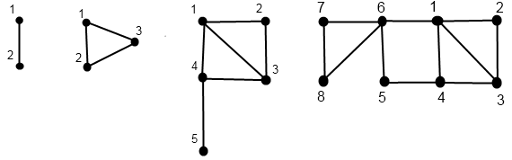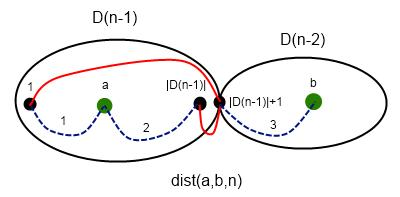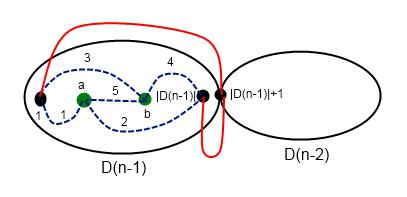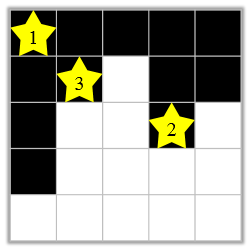# UOJ62「UR #5」怎样跑得更快

## 题目大意

$\sum_{j=1}^n\gcd(i,j)^c\times lcm(i,j)^d\times x_j=b_i \pmod P$

## 数据范围

$1 \le n \le 10^5$

## 解题思路

$\sum_{j=1}^n\gcd(i,j)^{c-d}\times \frac{x_j}{j^d}=\frac{b_i}{i^d} \pmod P\\ \sum_{j=1}^n\gcd(i,j)^{c-d} X_j=B_i \pmod P\\$

$\sum_{j=1}^nf(\gcd(i,j))X_j=B_i\\ \sum_{j=1}^n\sum_{d|i,d|j}f_r(d)X_j=B_i\\ \sum_{d|i}f_r(d)\sum_{j=kd}X_j=B_i\\ \sum_{d|i}f_r(d)S(d)=B_i\\$

# CF232C Doe Graphs

## 题目大意

• $D(0)$ 由节点 1 构成
• $D(1)$ 由节点 1, 2 和边 (1, 2) 构成
• $D(n)$ 以如下方式构造
• $D(n-2)$ 的中的点编号都加上 $|D(n-1)|$
• 在点 $|D(n-1)|$ 和点 $|D(n-1)|+1$ 之间连边。
• 在点 $|D(n-1)|+1$ 和点 1 之间连边## 数据范围

$1 \le n, q \le 10^5\\ 1 \le x ,y \le 10^{16}$

## 解题思路

### 情况一：a 在左部图，b 在右部图$Dis(a,b,n)=\min(Dis(1,a,n-1),Dis(a,|D(n-1)|,n-1))+Dis(1,B-|D(n-1)|,n-2)$

### 情况三：a，b 均在左部图$Dis(a,b,n)=\min\left\{ \begin{matrix} Dis(a,b,n-1)\\ Dis(1,a,n-1)+Dis(b,|D(n-1)|,n-1)+2\\ Dis(1,b,n-1)+Dis(a,|D(n-1)|,n-1)+2\\ \end{matrix}\right.$

# CF1310 Au Pont Rouge

## 题目大意

eg. $S=abaabb,m=3$，存在分割方案 $\{ab,aab,b\}$，则我们用字典序最小的 $aab$ 来代表这个分割方案。

## 数据范围

$2 \le n \le 1,000,1 \le m \le 1,000, 1 \le k \le 10^{18}$

## 解题思路

$dp[x][y] = d[t][y-1]\\ d[x][y] = dp[x][y]+d[x+1][y]$

## 题目大意

• 可以向上下左右四个方向走
• 碰到边界或者墙就停止，否则不可以停下

## 样例

3 7
#..O..#
#.###.#
*..#..*

NO

6 6
*..*##
..O...
*..*#.
####*.
......
.....#

YES


## 数据范围

$1 \le H,W \le 50$

## 解题思路

• 每个特殊点上下肯定在一个连通分量内，左右亦然，这个点被经过当且仅当这两个连通分量至少有一个被到达。
• 从出发点到不了的连通分量必不能到达
• 如果 A 和 B 两个连通分量没有祖先关系必不能同时到达。

# D. Yui and Mahjong Set

## 题目大意• 查询操作：将一个数 $x(1\le x\le n)$ 插入集合 $S$，然后交互器会告诉你插入结束后集合 $S$ 中的 $\mathbf{triplet}$$\mathbf{straight}$ 的数量。

• 回答操作：令 $c_i$ 表示集合 $S$ 中权值为 $c_i$ 的数的个数，则你需要求出对于初始的集合 $S$，求出 $c_1,c_2\sim c_n$

• 交互器会告诉您 $n$ 以及初始时集合 $S$ 中的 $\mathbf{triplet}$$\mathbf{straight}$ 的数量。

## 数据范围

$4\le n\le 100$

# UOJ507「JOISC2020」星座3

## 题目大意

$JOI-kun$ 拍了一张夜景照片。这张照片由 $N \times N$ 个像素组成，即长宽均为 $N$ 个像素。从左到右第 $x$ 列，从上往下第 $y$ 行的像素被称为 $(x,y)$

• 矩形区域中没有白色像素。
• 矩形区域中有两个或多个黄色像素。

$JOI-kun$ 已经厌倦了看星座。因此他想把一些黄色像素画成黑色，使得修改后的照片不存在任何星座。

## 样例

Input:
5
1 3 4 2 3
3
1 5 3
4 3 2
2 4 2

Output:
2## 解题思路

• $S \ge C$ 答案直接加上 $C$，因为在上面的点更容易和别的点冲突，而且代价更大，肯定不优，删掉
• $S < C$ 答案暂时加上 $S$，并将区间 $[L, R]$ （表示能够到达区域）每个值加上 $C - S$

posted @ 2021-05-25 07:38  Hs-black  阅读(228)  评论(0编辑  收藏  举报